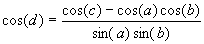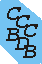Computational Chemistry Comparison and Benchmark DataBase Release 22 (May 2022) Standard Reference Database 101 National Institute of Standards and Technology Home All data for one species Geometry Experimental Calculated Comparisons Bad Calculations Tutorials and Explanations Vibrations Experimental Calculated Scale factors Reactions Entropies Ions List Ions Energy Electron Affinity Proton Affinity Ionization changes point group Experimental One molecule all properties One property a few molecules Geometry Vibrations Energy Electrostatics Reference Data Calculated Energy Optimized Reaction Internal Rotation Orbital Nuclear repulsion energy Correlation Ion Excited State Basis Set Extrapolation Geometry Vibrations Frequencies Zero point energy (ZPE) Scale Factors Bad Calculations Electrostatics Charges Dipole Quadrupole Polarizability Spin Entropy and Heat Capacity Reaction Lookup by property Comparisons Geometry Vibrations Energy Entropy Electrostatics Ion Resources Info on Results Calculations Done Basis functions used I/O files Glossary Conversion Forms Links NIST Links External links Thermochemistry Tutorials Vibrations Entropy Energy Electrostatics Geometry Cost Bad Calculations FAQ Help Units Choose Units Explanations Credits Just show me Summary Using List Recent molecules Molecules Geometry Vibrations Energy Similar molecules Ions, Dipoles, etc. Index of CCCBDB Feedback You are here: Home > Geometry > Tutorials and Explanations > Angle formulas OR Resources > Conversion Forms > Angle formulas OR Resources > Tutorials > Geometry > Angle formulas

# Notes on converting between angles in tetravalent centers

## C3V moleculesIn a C3v molecule such as CH3F, the angles FCH and HCH are related by symmetry. The angles a (FCH) and b (HCH) are related using the following formulas: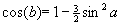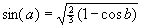Please note that the latter solution is not unique, and that solving for angle a may return 180° - a enter an angle greater than 0 and less than 180 a= Click to solve for b enter an angle greater than 0 and less than 120 b= Click to solve for a Click to clear

## C2V pentatomic molecules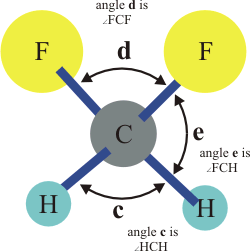In a C2V molecule such as CH2F2 the angle HCF can be related to the two angles HCH and FCF by symmetry. The angle e (HCF) is related to the angles c (HCH) and d (FCF) by: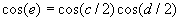Please note that the latter solution is not unique, and that solving for angle e may return 180° - e enter two angles between 0 and 180 degrees c= d= e= Solve for angle c Solve for angle d Solve for angle e Clear

## Cs tetravalent molecules

There are six possible angles, but only three are needed to define the tetravalent center. Given three angles what is the fourth?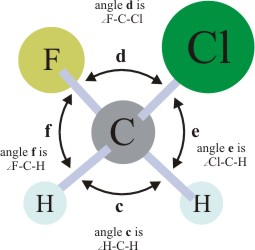We use fluorochloromethane as an example. We know: angle c (H-C-H) (there is only one) angle d (F-C-Cl) (there is only one) angle e (H-C-Cl) (there are two equivilant) We want angle f (F-C-H) (there are two equivilant)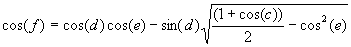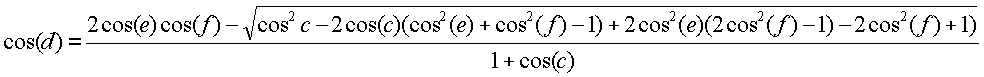## Arbitrary tetravalent center

Given five of the possible angles what is the sixth?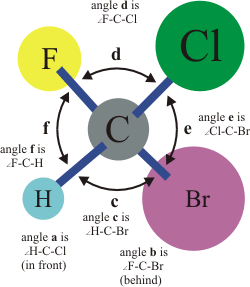In a arbitrary tetravalent center their are six angles. We define these angles as: a is angle H-C-Cl b is angle F-C-Br c is angle H-C-Br d is angle F-C-Cl e is angle Cl-C-Br f is angle H-C-F Assume we want angle f as a function of the other five angles. We can work with the cosines of the angles: ca = cos(a), cb = cos(b),etc. Define p and q: p = sqrt( (ca2 + cc2 + ce2 - 2*ca*cc*ce - 1) * ((ca2 + cb2 + cd2 - 2*ca*cb*cd - 1) ) q = ca*(cb*ce + cc*cd) - cb*cc - cd*ce The two solutions for cos(f) are: cf = ( p + q)/(ca2 - 1) cf = (-p + q)/(ca2 - 1)

## Dihedral from three angles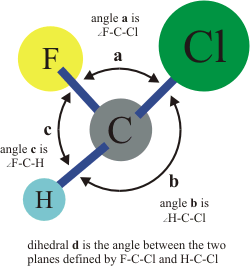Given the three angles: a is angle F-C-Cl b is angle H-C-Cl c is angle H-C-F We want the dihedral angle d (the angle between the two planes defined by F-C-Cl and H-C-Cl).